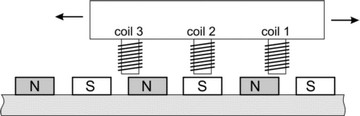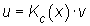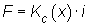﻿ 20-sim webhelp > Toolboxes > Mechatronics Toolbox > Servo Motor Editor > Theory > Basic Principles > Linear Motors

# Linear Motors

Navigation:  Toolboxes > Mechatronics Toolbox > Servo Motor Editor > Theory > Basic Principles >

# Linear MotorsThe working principle of linear motors is exactly the same as for rotary motors. Magnets are place on a flat surface, as shown in the figure below. A carriage holding the coils can run over the magnets.When the carriage runs over the magnets an electromotive voltage will be induced:with Kc(x) the force function of the coil. It is a function of the carriage position, shape of the coil, magnetic field density, current distribution etc. The force function in a linear motor is equivalent to the torque function of a rotary motor. When a current runs through the coil a force results:The principle for linear motor is the same as the principle of rotary motors. By a proper design of the motor, i.e. geometry of the coil windings, magnets etc., the force function Kc(x) can be given a particular shape. By proper manipulation of the current a positive force can be created during the movement of the carriage. Because mechanical commutation is not very useful for linear movements, linear motors use electronic commutation. Like their rotary counterparts, linear motors can be driven by block shape currents (Brushless DC Linear) and sinusoidal currents (AC Synchronous Linear).

## Limits

The limits for linear motors are equal to the Brushless DC and AC synchronous motors when torque is replaced by force and angular speed by linear speed.